## Excel IF: The Ultimate Guide# Excel IF: The Ultimate Guide

### Introduction

Let me start by saying that the IF Statements in Excel is my favorite Excel Function of all time! It is the purest form of Logic and in order to take our work to the next level, we need to be able to build logic.

### Why is this Function so Important?

Briefly explaining, the IF function Excel performs a user instructed logical test, if that test is TRUE, it performs an action, if it is FALSE, it does another action. These actions can do many things, from displaying text to performing a simple calculation or even other logical IF tests.

Now, my favorite way to explain the concept of the IF Formula Excel is to talk about the film: The Matrix!

In the most pivotal scene of the film, the main character Neo is faced with a dilemma, he can consume a Blue colored pill and continue to remain in the Matrix and live his normal life OR he can take the Red pill, leave the Matrix and see how far down the rabbit hole he can go.

We made the following tree diagram to explain this:What this tree diagram is saying is, if he takes the Red Pill, he wakes up. But if he doesn’t take the Red Pill he stays asleep. Now, let me just explain the syntax of the IF statements in Excel, then I’ll come back to this.

#### Syntax of the Function Explained

=IF(logical_test,[value_if_true],[value_if_false])

This function is one of the most useful in Excel.  When harnessed and nested (using multiple IF functions in one), you can have one very powerful multi levelled formula in ONE cell that performs an astonishing number of logical tests.  First, let’s get to grips with the dissecting the syntax above for a single IF function.

logical_test

As per Excel, it defines this part of the syntax as “any value or expression that can be evaluated to TRUE or FALSE.”.  This is where you make your test of a cell, see below under Examples for more detail.

[value_if_true]

As per Excel, it defines this as “the value that’s returned if Logical_test is TRUE, if omitted, TRUE is returned.  You can nest up to seven IF functions”

A quick note on the above,  it’s important to know that you CAN nest over and above seven IF functions.  There is a limit of 7 nested functions, but only up to Excel 2007.  Since then, it’s been increased to 64 IF statements in ONE formula.  I’ve yet to get there!

[value_if_false]

As per Excel, it defines this as “the value that’s returned if Logical_test is FALSE, if omitted, FALSE is returned.

Ok, let’s start with a very basic non-real-world example.

##### Examples

Basic IF

Try replicating the below.  Write our IF function in cell C2 to test cell B2 to see if it equals 10, the function will perform this logical test for us, if it is TRUE, then display the text Yes, if it FALSE, then display No.

In the function below, the part B2=10 is our logical test, the text, Yes, is our result if the logical test above is TRUE and the text, No, is our result if the logical test is FALSE.

Remember, when asking a function to display text, you must enclose the text with quotation marks.This basic IF function not only works with numbers, it also works with text too.In the example above we are testing cell B4 to see if it equals test, and displaying yes when the logical test if found to be TRUE, and no, when FALSE.

Just to prove the function works for BOTH TRUE and FALSE, cell B5 is spelt incorrectly, so the function finds this to be FALSE, therefore returning a no result.

Got it, ok great!  Let’s go back to my Matrix example!

So, if we put Neo’s dilemma in to the form of a formula, we get this:

=IF(Neo Takes Red Pill, “Go in to the Matrix”, “Go Home”)

Now, I won’t spoil it for you but what this is, is a logical test.

So, let’s look at that:

#### Understanding Logical Tests

The logical test part of the function can be used to do MANY different types of tests.  Think of it as a question about the cell you are wanting to analyse and ask yourself what you want to check, in all likelihood the question you have can be applied into a logical statement.

Typical logical tests you can do can be achieved by using the following symbols.  We’ve already looked at the equals sign (=), so others to think about are bLet’s look at some of these in examples by using a sample set of data below.

##### Greater ThanIn the example data set above (and we’ll continue to use this data set throughout and using columns C to I), we have a sales history for the last year.  In Column A we have the Months, and Column B, the sales in £’s.

In the cell C2, we have posed a question about the sales history.  Imagine you wanted to find out if the performance for that month meets an expectation, you can show this by including your threshold of £100 into the equation.

In the example above in we’ve set a logical test in cell C4 to check that when sales are GREATER than £100, show ‘Target Achieved’.  The logical test GREATER THAN is using the symbol shown in C3 (all symbols for the remainder of the examples will be here on this row).

Once the function is completed you can click on the fill handle (Green square on the bottom right of cell C4 above) and drag it down as far as you need to.  This will apply the same function to each cell and as a result it’ll find this logical test to be TRUE on rows 4, 6, 11 and 14.

You’ll see in row 7, we have £100 sales so the result finds the logical test to be FALSE as we said GREATER THAN £100.  We’ve then asked the function to display BLANK when the logical test is FALSE, and in order to do that you can do double quotations (2 of these “).

Less ThanVery similar to the above, but reversed logic.

Above we found in column for rows 4, 6, 11 and 14 to be TRUE.  On this occasion, they are now the FALSE part of the logic.  Note than C7 is also FALSE.  I think you know why, don’t you?

Is £100 Less than £100?

No, so the function will return the FALSE value, and in this instance, we’ve asked it to display BLANK.

Are you ready for more? Excellent! Get ready for another notch up in tempo? Brilliant, I like your enthusiasm! Let’s have a look at two very similar logical tests!

Greater Than or Equal To & Less Than or Equal ToColumn E: Imagine you only received a 10% commission on sales when hitting a target value or above?  You can do this by setting your logical test as above, clear?  Let’s walk through the logic and use row 4 as the example.

=IF(B4>=100,B4*10%,””)

By now you can probably work this out but for completeness, Excel will evaluate the above as per below:

=IF(150>100,150*10%,””)

The logical test above in bold is TRUE, so it will now process the next part of the syntax as 10% of 150, which comes to 15.

=IF(TRUE,15,””)

The above is now evaluated to ask IF TRUE, then show 15, else “”

Column F: As above, and again, reverse the logic, cell F4 would read =IF(B4<=100,B4*10%,””), or are

sales less than or equal to 100, if so calculate 10% of sales, otherwise show BLANK.

Going up one more notch below.

Does NOT EqualRemember from Mathematics class the equals sign with a cross through it, well <> is Excels answer to that!

This can be used in many ways, you can check if a cell equals a certain number. Imagine however, you want to indicate which month is the current one?   In the example above I’ve asked the function to display Current Month when a FALSE result is found when asked, does the month found in column A match the month entered in cell G18.

Note: The cell reference G18 is absolute as it has the dollar signs in front of the column and row reference, thus locking it in position when using fill handles to copy vertically or horizontally.

Last one, and a double!

ISTEXT & ISNUMBER

Typing these in as independent formulas will yield a TRUE or FALSE result, give it a go, enter the number 3 into cell A1 and then type in A2 =ISNUMBER(A1), you get TRUE.  Same can be said for typing TEST in A1 and ISTEXT.This example is all text based and as per the written example above you can make some checks to data to ensure your worksheets are complete and alert a user to missing data.

In this case we’ve alerted the user that the month is missing in A10 and there are no sales entered in B8.  This can be very useful to get worksheets to do the thinking for you.

Remember, you can enter anything you want into the TRUE and FALSE part of the above, the choice IS yours!

Less thinking by you = more time to learn other new skills on Excel, and repeat = Excel genius!

##### The Excel IF OR Function

What does it do?

Similar to IF AND, the Excel IF OR function is a combination of two separate functions.  When combined they perform a multiple user instructed logical test, if any ONE or more of those tests are TRUE, it performs an action, if ALL of the tests are FALSE, it does another action.  These actions can many things, from displaying text, performing a simple calculation.

Ok, let’s start with a very basic example.

Examples

As we did with IF AND, let’s first see what the OR function does on its own.  In summary, it checks if ANY one argument is TRUE and then returns TRUE, otherwise it returns FALSE.Above we have a selection of fruit and veg., in column C we can test column B to see if it contains the text Carrots or Cabbage.  Cells C4 and C7 are both TRUE, since B4 and B7 contained Carrots OR Cabbage.  Pretty simple right.

Evaluating the above (In the toolbar, select FORMULAS > EVALUATE FORMULA) we can see a step by step calculation of each argument:Use this tool to help you spot errors in the logic, it’ll save you time and energy in the long run.  Ok, let’s get into the IF OR combined, see below.

#### Basic IF OR

We can adapt the above by taking the OR function (our logical test):

OR(B4=”Carrots”,B4=”Cabbage”)

And inserting it into the IF function shown in red:

IF(logical_test,[value_if_true],[value_if_false])

IF(OR(B4=”Carrots”,B4=”Cabbage”),[value_if_true],[value_if_false])

So now all is left to do is to instruct the IF function to do THIS for TRUE and do THAT for FALSE.

This gives us the below:

=IF(OR(B4=”Carrots”,B4=”Cabbage”),”Vegetable”,”Fruit”)

Remember, when asking a function to display text, you must enclose the text with quotation marks.Above is how it looks in Excel, be mindful when working with text, it can cause you some headaches.  Let’s quickly look at ways this can go wrong, understanding these now can save you time down the road.

Ok, same example above, but can you spot the mistake?

Mistake 1If you look closely you can see that the word “Carrots” is spelt incorrectly as “Carots”.  Excel isn’t clever enough to spot these typos, you MUST match exactly.  Now, look at the next one, this is VERY TRICKY!

Mistake 2Without actually having Excel to look at all the data it isn’t obvious, in fact it’s impossible.  The formula looks correct, right?  Yes, it is, but what you cannot see is this in cell B4 and B7!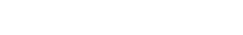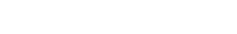Can you spot it!?  Notice the cursor is away from the text, that’s because there is a [space] in front or at the end of the text, so again, Excel isn’t smart enough to spot these, so your formula WILL fail.  Ways around this are to edit the data manually, or use the TRIM function, very simple to use, barely requires any explanation.

Let’s move on to another example

##### Basic IF OR with SUMAbove we have a cost analysis of trip being arranged for 5 students, rows 11 and 12 list the flat rates per student.  All students are attending and only 4 of the 5 students are taking a lunch.  Each student can choose between Hot, Cold or Vegetarian Lunch.

With cell E15 selected you can see the OR function in the red box, here we test D15 if any of the 3 options appear, being careful that our spelling is right.

The blue box is the TRUE action if ANY of the logical_tests above are TRUE.  It’s here we can instruct it to perform a SUM calculation, above we’ve totalled Seminar Base Cost + Lunch = £107.50.

The green box is the FALSE action if ALL of the logical_tests above are FALSE.  Here we’ve told it to only consider the Seminar Base Cost since none of the options were chosen, see cell D18.

#### Written down this IF OR is asking:

IF the student has either Hot, Cold OR Vegetarian, then add Seminar Base Cost and Lunch Fees, otherwise just show Seminar Base Cost.

Remember, the above only covers a few logical tests in the OR function, you have a limit of up to 255!  The possibilities are endless!

### What does it do?

Similar to IF AND, and IF OR, the Excel IF OR AND function is a combination of three separate functions.  When combined they can perform a very useful multiple user instructed logical test.  Note it doesn’t have to be this order, the great thing about this trio is the can be mixed, nested and intertwined!

For the OR function, we know that if any ONE or more of those tests are TRUE, it performs an action, if ALL of the tests are FALSE, it does another action.

For the AND function, we know that if ALL of those tests are TRUE, it performs an action, if ALL of the tests are FALSE, it does another action.

These actions can many things, from displaying text, performing a simple calculation or getting straight into another OR or AND function.  You can get very deep into the rabbit hole but let’s take it easy to begin with.

Ok, let’s start with a very basic example.

#### Examples

Ok, let’s keep this simple for now, see below example, best to copy this out so you can understand fully.Above we’re going to test the data in Row 3 and ask:

If the value in B3 = 50 AND C3 = 100 OR the value D3 = Blue AND E3 = Green, then show TRUE

The OR part is key here, we only need one side to be TRUE.

Start your IF function, and you’ll see the part in bold prompts you to enter the logical.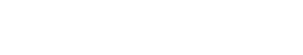Staying inside the logical_test, type OR( to perform an OR test, again it prompts you to state your logical1 of the OR function.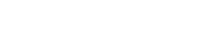Staying inside the logical1 of the OR function and the logical_test of the IF function, we can now move into the AND function, of which prompts us for the logical1 part of the AND.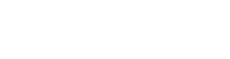Start the AND test as per above, then close off the AND function with a parenthesis and it’ll take you back to the logical1 part of the OR function, you can now press the comma key to move to logical2 of the OR function.Finish the next AND test off as per above and close with a parenthesis, you can now see the yellow comment box underneath the OR function, and in bold it is the logical2 part of the OR function. We can now close off the original OR function with another parenthesis.

All this time we have been inside the logical_test part of the IF function Excel. It’s tricky I know but with practice and over time you can make some ridiculously complex creations! Once you grasp the basics you’ll want to try out more, it’ll make your workbooks do a lot of thinking for you.Ok, finally add the TRUE and FALSE into the last part (Excel allows this text without quotations marks, good when building your conditions from scratch to test them), we knew it would be TRUE because we’ve asked does B3 = 50 AND C3 = 100 (yes it does) OR does D3 = Blue AND E3 = Green (also yes).Let’s adjust some of the values and apply the same formula vertically in the results column.

Above you’ll see the results down Column H.  See if you can spot why some are FALSE and others are TRUE.  I’ve colour mapped the numbers and colours for ease.

TRUE results will have BOTH TRUE on Value 1 AND Value 2 OR BOTH TRUE on Value 3 AND Value 4.  So, in the above we can see this will be TRUE for rows 3, 5, 6, 8 and 9 as they have 50 AND 100 OR Blue AND Green on their respective columns.

The opposite can be found on row 4 and 7.  Although row 4 has 100 in C4, is doesn’t have the 50 in B4, same to be said for the colours, it can find a positive match for Green in F4 but is missing the Blue in E4. Row 7 just cannot match anything.

Remember to watch out for mis-spellings, in the cells, formulas and those rogue spaces in your text.  These functions do not mind if you use capitals, but try to match the text casing exactly, it just makes for good practise and ease of reading

Let’s take a look at swapping AND with OR and vice versa.  Because AND and OR work in the same format of logical1, logical 2 etc., you can literally change as per below.  Note: As I’ve worked with a duplicate table the cells refs are changed, so if you want to mirror identically you can copy your table beneath the original.With the same data set you get the below:Analysing the above you’ll see the results down Column H again.  See if you can spot why some are FALSE and others are TRUE.  The data hasn’t changed, just the formula we built in Column H.

TRUE results will have at least one TRUE on Value 1 OR Value 2 AND at least one TRUE on Value 3 OR Value 4.  So, in the above we can see this will be TRUE for rows 15, 16, 17, 18 and 22 as they have 50 OR 100 AND Blue OR Green on their respective columns.

The opposite can be found on row 19, 20 and 21.  Although row 20 has 100 in C20, is doesn’t have the Blue or Green on the other side.  Row 21 has at least one with Blue in B21 OR Green in F21, but fails to have at least one TRUE result in the numbers section with 40 and 90.  Row 19 just cannot match anything so returns FALSE.

Now, you may nest to your hearts content…. Almost!  It will limit up to 255 OR and AND statements combined.